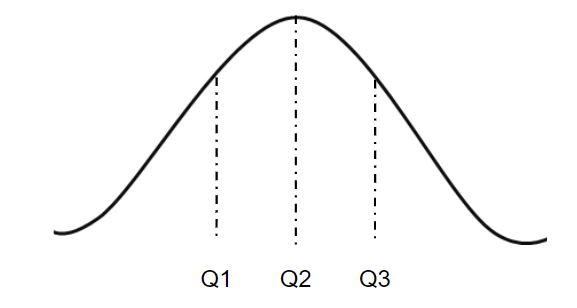# numpy.nanquantile() in Python

`numpy.nanquantile(arr, q, axis = None)` : Compute the qth quantile of the given data (array elements) along the specified axis, ignoring the nan values.

Quantile plays a very important role in Statistics when one deals with the Normal Distribution.In the figure given above, `Q2` is the `median` of the normally distributed data. `Q3 - Q2` represents the Interquantile Range of the given dataset.

Parameters :
arr : [array_like]input array.
q : quantile value.
axis : [int or tuples of int]axis along which we want to calculate the quantile value. Otherwise, it will consider arr to be flattened(works on all the axis). axis = 0 means along the column and axis = 1 means working along the row.
out : [ndarray, optional]Different array in which we want to place the result. The array must have same dimensions as expected output.

Results : qth quantile of the array (a scalar value if axis is none) or array with quantile values along specified axis, ignoring nan values.

Code #1 :

 `# Python Program illustrating  ` `# numpy.nanquantile() method   ` `import` `numpy as np  ` `     `  `# 1D array  ` `arr ``=` `[``20``, ``2``, ``7``, np.nan, ``34``]  ` `print``(``"arr : "``, arr)  ` ` `  `print``(``"\n-Q1 quantile of arr : "``, np.quantile(arr, .``50``))  ` `print``(``"Q2 - quantile of arr : "``, np.quantile(arr, .``25``))  ` `print``(``"Q3 - quantile of arr : "``, np.quantile(arr, .``75``))  ` ` `  `print``(``"\nQ1 - nanquantile of arr : "``, np.nanquantile(arr, .``50``))  ` `print``(``"Q2 - nanquantile of arr : "``, np.nanquantile(arr, .``25``))  ` `print``(``"Q3 - nanquantile of arr : "``, np.nanquantile(arr, .``75``))  `

Output :

```arr : [20, 2, 7, nan, 34]

Q1 - quantile of arr : nan
Q2 - quantile of arr : nan
Q3 - quantile of arr : nan

Q1 - nanquantile of arr : 13.5
Q2 - nanquantile of arr : 5.75
Q3 - nanquantile of arr : 23.5
```

Code #2:

 `# Python Program illustrating  ` `# numpy.nanquantile() method  ` ` `  `import` `numpy as np  ` ` `  `# 2D array  ` `arr ``=` `[[``14``, np.nan, ``12``, ``33``, ``44``],  ` `       ``[``15``, np.nan, ``27``, ``8``, ``19``],  ` `       ``[``23``, ``2``, np.nan, ``1``, ``4``, ]]  ` `print``(``"\narr : \n"``, arr)  ` `     `  `# quantile of the flattened array  ` `print``(``"\nQ2 quantile of arr, axis = None : "``, np.quantile(arr, .``50``))  ` `print``(``"\nQ2 quantile of arr, axis = None : "``, np.nanquantile(arr, .``50``))  ` `print``(``"0th quantile of arr, axis = None : "``, np.nanquantile(arr, ``0``))  `

Output:

```arr :
[[14, nan, 12, 33, 44], [15, nan, 27, 8, 19], [23, 2, nan, 1, 4]]

Q2 quantile of arr, axis = None : nan
Q2 quantile of arr, axis = None : 14.5
0th quantile of arr, axis = None : 1.0
```

Code #3:

 `# Python Program illustrating  ` `# numpy.nanquantile() method  ` `import` `numpy as np  ` ` `  `# 2D array  ` `arr ``=` `[[``14``, np.nan, ``12``, ``33``, ``44``],  ` `    ``[``15``, np.nan, ``27``, ``8``, ``19``],  ` `    ``[``23``, ``2``, np.nan, ``1``, ``4``, ]]  ` `print``(``"\narr : \n"``, arr)  ` `         `  `# quantile along the axis = 0  ` `print``(``"\nQ2 quantile of arr, axis = 0 : "``, np.nanquantile(arr, .``50``, axis ``=` `0``))  ` `print``(``"0th quantile of arr, axis = 0 : "``, np.nanquantile(arr, ``0``, axis ``=` `0``))  ` ` `  `# quantile along the axis = 1  ` `print``(``"\nQ2 quantile of arr, axis = 1 : "``, np.nanquantile(arr, .``50``, axis ``=` `1``))  ` `print``(``"0th quantile of arr, axis = 1 : "``, np.nanquantile(arr, ``0``, axis ``=` `1``))  ` ` `  `print``(``"\nQ2 quantile of arr, axis = 1 : \n"``, ` `  ``np.nanquantile(arr, .``50``, axis ``=` `1``, keepdims ``=` `True``))  ` `print``(``"\n0th quantile of arr, axis = 1 : \n"``, ` `    ``np.nanquantile(arr, ``0``, axis ``=` `1``, keepdims ``=` `True``))  `

Output:

```arr :
[[14, nan, 12, 33, 44], [15, nan, 27, 8, 19], [23, 2, nan, 1, 4]]

Q2 quantile of arr, axis = 0 : [15.  2. 19.5  8.  19. ]
0th quantile of arr, axis = 0 : [14. 2. 12.  1.  4.]

Q2 quantile of arr, axis = 1 : [23.5 17.   3. ]
0th quantile of arr, axis = 1 : [12.  8.  1.]

Q2 quantile of arr, axis = 1 :
[[23.5]
[17. ]
[ 3. ]]

0th quantile of arr, axis = 1 :
[[12.]
[ 8.]
[ 1.]]```

My Personal Notes arrow_drop_upIf you like GeeksforGeeks and would like to contribute, you can also write an article using contribute.geeksforgeeks.org or mail your article to contribute@geeksforgeeks.org. See your article appearing on the GeeksforGeeks main page and help other Geeks.

Please Improve this article if you find anything incorrect by clicking on the "Improve Article" button below.

Article Tags :

Be the First to upvote.

Please write to us at contribute@geeksforgeeks.org to report any issue with the above content.